# 6421591

Оценка 4.8
ppt
08.05.2020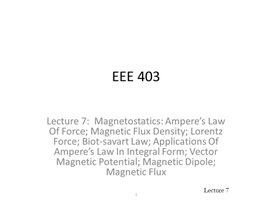6421591.ppt

EEE 403

Lecture 7: Magnetostatics: Ampere’s Law Of Force; Magnetic Flux Density; Lorentz Force; Biot-savart Law; Applications Of Ampere’s Law In Integral Form; Vector Magnetic Potential; Magnetic Dipole; Magnetic Flux

1

## Lecture 7 Objectives To begin our study of magnetostatics with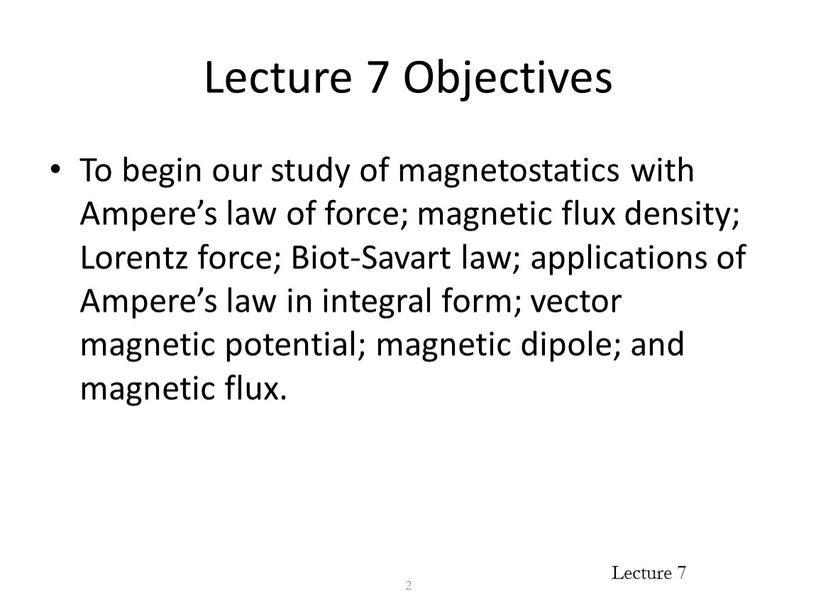Lecture 7 Objectives

To begin our study of magnetostatics with Ampere’s law of force; magnetic flux density; Lorentz force; Biot-Savart law; applications of Ampere’s law in integral form; vector magnetic potential; magnetic dipole; and magnetic flux.

2

Overview of Electromagnetics

3

Maxwell’s
equations

Fundamental laws of classical electromagnetics

Special cases

Electro-statics

Magneto-statics

Electro-magnetic waves

Kirchoff’s Laws

Statics:

Geometric Optics

Transmission
Line
Theory

Input from other disciplines

## Magnetostatics Magnetostatics is the branch of electromagnetics dealing with the effects of electric charges in steady motion (i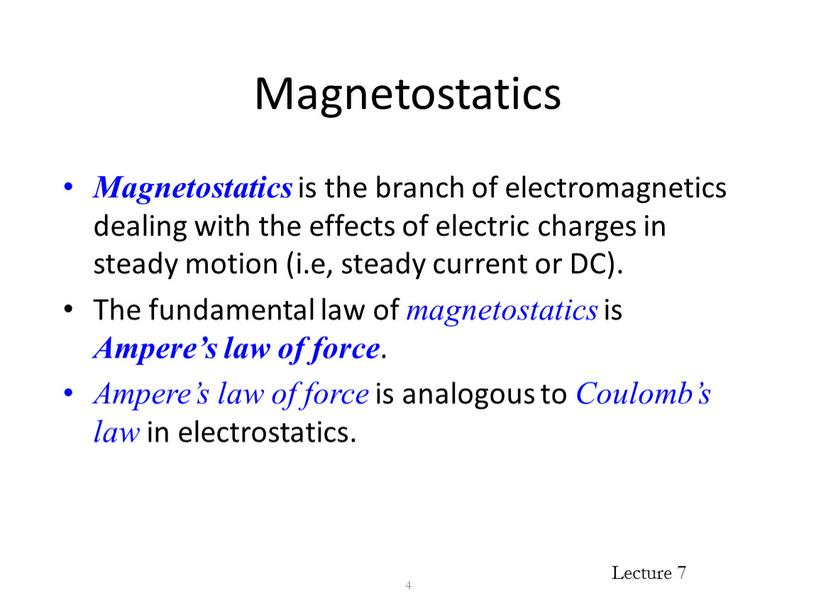Magnetostatics

Magnetostatics is the branch of electromagnetics dealing with the effects of electric charges in steady motion (i.e, steady current or DC).
The fundamental law of magnetostatics is Ampere’s law of force.
Ampere’s law of force is analogous to Coulomb’s law in electrostatics.

4

## Magnetostatics (Cont’d) In magnetostatics, the magnetic field is produced by steady currents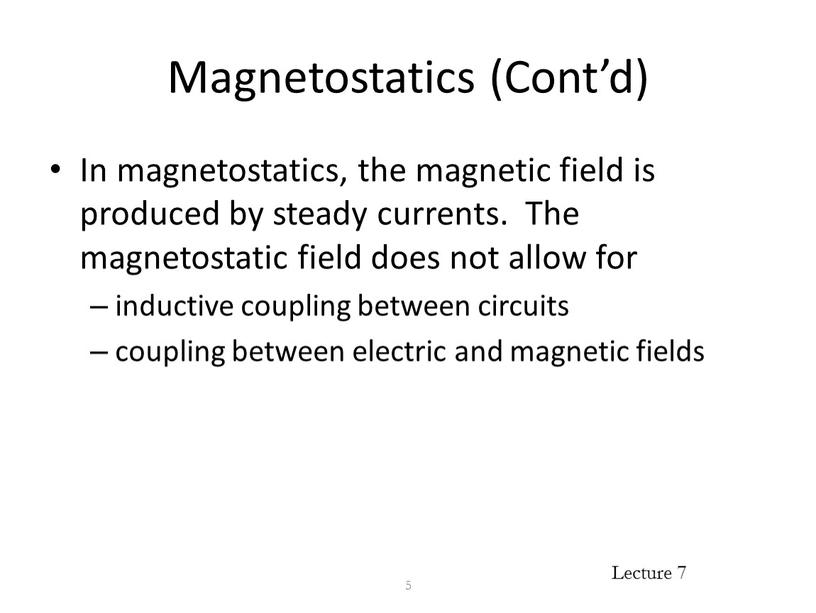Magnetostatics (Cont’d)

In magnetostatics, the magnetic field is produced by steady currents. The magnetostatic field does not allow for
inductive coupling between circuits
coupling between electric and magnetic fields

5

## Ampere’s Law of Force Ampere’s law of force is the “law of action” between current carrying circuits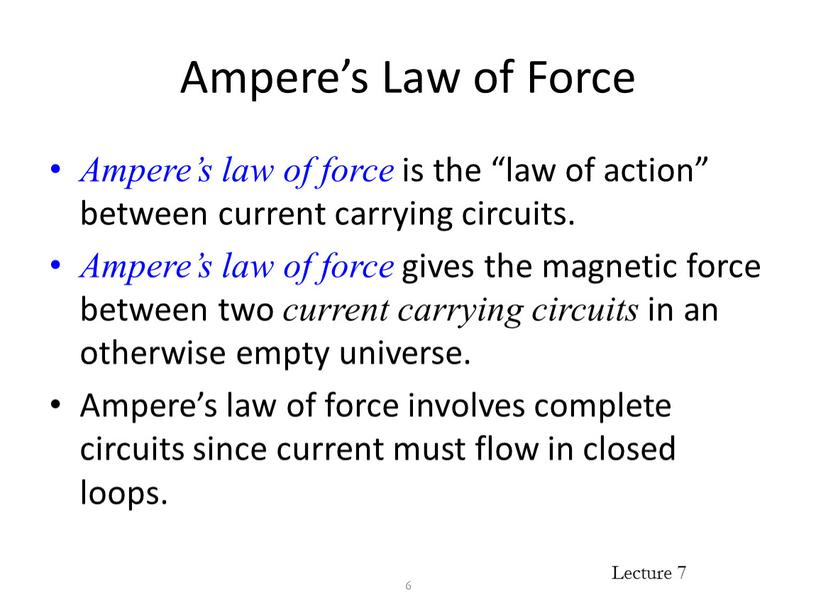Ampere’s Law of Force

Ampere’s law of force is the “law of action” between current carrying circuits.
Ampere’s law of force gives the magnetic force between two current carrying circuits in an otherwise empty universe.
Ampere’s law of force involves complete circuits since current must flow in closed loops.

6

Ampere’s Law of Force (Cont’d)

Experimental facts:
Two parallel wires carrying current in the same direction attract.
Two parallel wires carrying current in the opposite directions repel.

7

Ampere’s Law of Force (Cont’d)

Experimental facts:
A short current-carrying wire oriented perpendicular to a long current-carrying wire experiences no force.

8

I1

F12 = 0

I2

Ampere’s Law of Force (Cont’d)

Experimental facts:
The magnitude of the force is inversely proportional to the distance squared.
The magnitude of the force is proportional to the product of the currents carried by the two wires.

9

Ampere’s Law of Force (Cont’d)

The direction of the force established by the experimental facts can be mathematically represented by

10

unit vector in direction of force on I2 due to I1

unit vector in direction of I2 from I1

unit vector in direction of current I1

unit vector in direction of current I2

Ampere’s Law of Force (Cont’d)

The force acting on a current element I2 dl2 by a current element I1 dl1 is given by

11

Permeability of free space
m0 = 4p  10-7 F/m

Ampere’s Law of Force (Cont’d)

The total force acting on a circuit C2 having a current I2 by a circuit C1 having current I1 is given by

12

Ampere’s Law of Force (Cont’d)

The force on C1 due to C2 is equal in magnitude but opposite in direction to the force on C2 due to C1.

13

## Magnetic Flux Density Ampere’s force law describes an “action at a distance” analogous to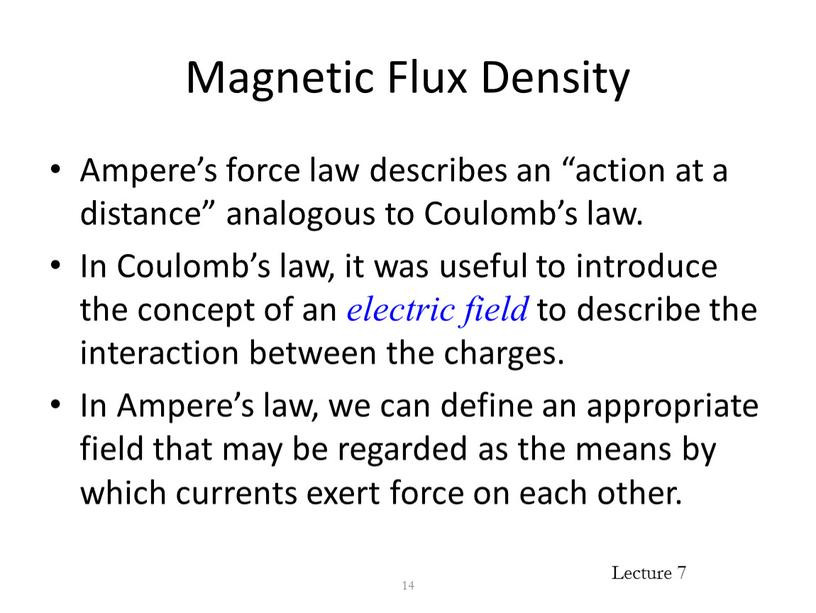Magnetic Flux Density

Ampere’s force law describes an “action at a distance” analogous to Coulomb’s law.
In Coulomb’s law, it was useful to introduce the concept of an electric field to describe the interaction between the charges.
In Ampere’s law, we can define an appropriate field that may be regarded as the means by which currents exert force on each other.

14

Magnetic Flux Density (Cont’d)

The magnetic flux density can be introduced by writing

15

## Magnetic Flux Density (Cont’d) where 16 the magnetic flux density at the location of d l 2 due to the current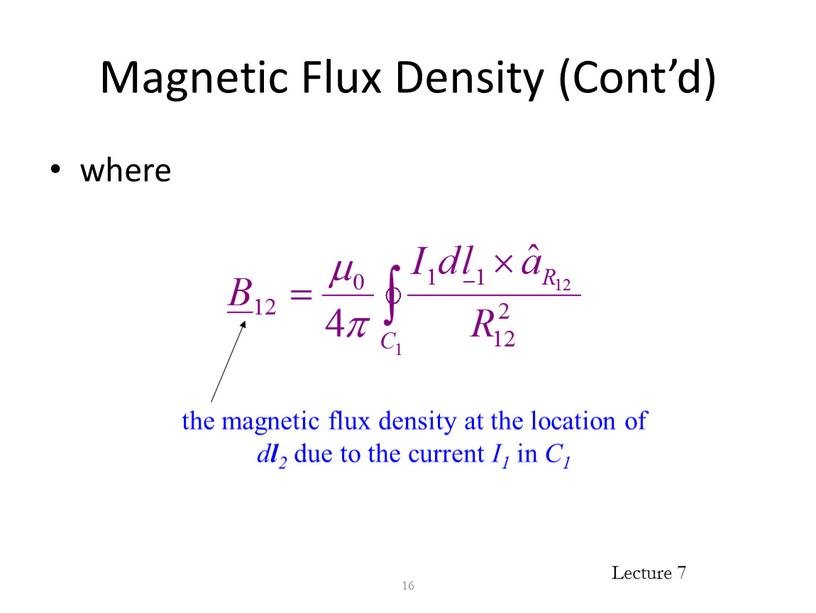Magnetic Flux Density (Cont’d)

where

16

the magnetic flux density at the location of dl2 due to the current I1 in C1

Magnetic Flux Density (Cont’d)

Suppose that an infinitesimal current element Idl is immersed in a region of magnetic flux density B. The current element experiences a force dF given by

17

Magnetic Flux Density (Cont’d)

The total force exerted on a circuit C carrying current I that is immersed in a magnetic flux density B is given by

18

## Force on a Moving Charge A moving point charge placed in a magnetic field experiences a force given by 19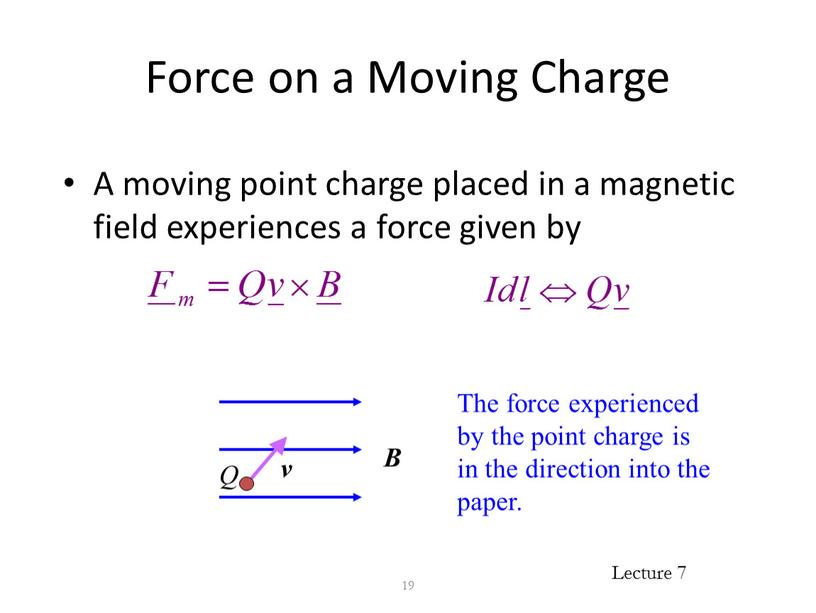Force on a Moving Charge

A moving point charge placed in a magnetic field experiences a force given by

19

The force experienced by the point charge is in the direction into the paper.

## Lorentz Force If a point charge is moving in a region where both electric and magnetic fields exist, then it experiences a total force given…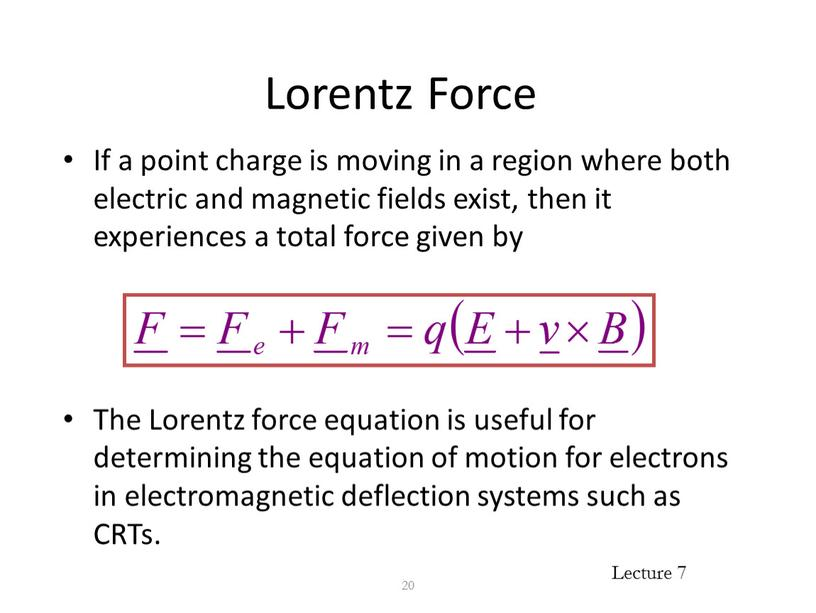Lorentz Force

If a point charge is moving in a region where both electric and magnetic fields exist, then it experiences a total force given by

The Lorentz force equation is useful for determining the equation of motion for electrons in electromagnetic deflection systems such as CRTs.

20

## The Biot-Savart Law The Biot-Savart law gives us the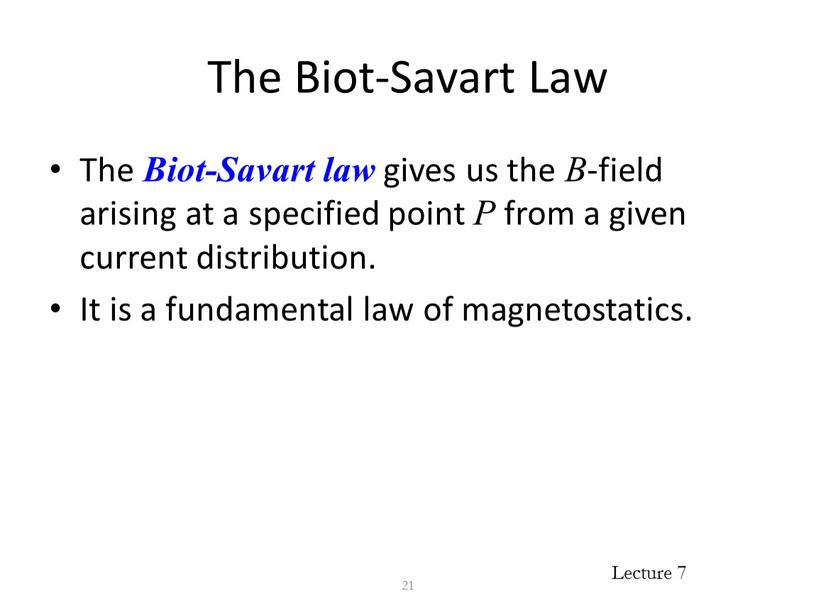The Biot-Savart Law

The Biot-Savart law gives us the B-field arising at a specified point P from a given current distribution.
It is a fundamental law of magnetostatics.

21

## The Biot-Savart Law (Cont’d) The contribution to the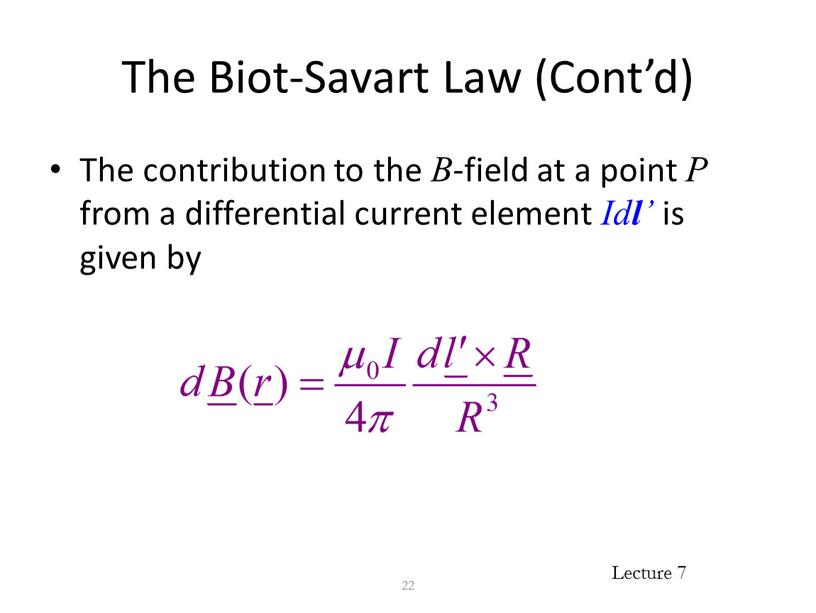The Biot-Savart Law (Cont’d)

The contribution to the B-field at a point P from a differential current element Idl is given by

22

The Biot-Savart Law (Cont’d)

23

## The Biot-Savart Law (Cont’d) The total magnetic flux at the point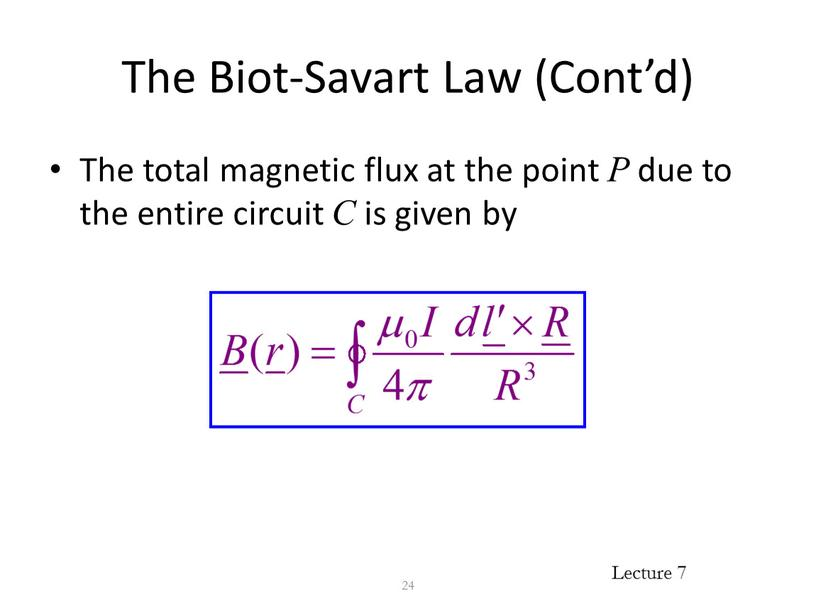The Biot-Savart Law (Cont’d)

The total magnetic flux at the point P due to the entire circuit C is given by

24

Types of Current Distributions

Line current density (current) - occurs for infinitesimally thin filamentary bodies (i.e., wires of negligible diameter).
Surface current density (current per unit width) - occurs when body is perfectly conducting.
Volume current density (current per unit cross sectional area) - most general.

25

## The Biot-Savart Law (Cont’d) For a surface distribution of current, the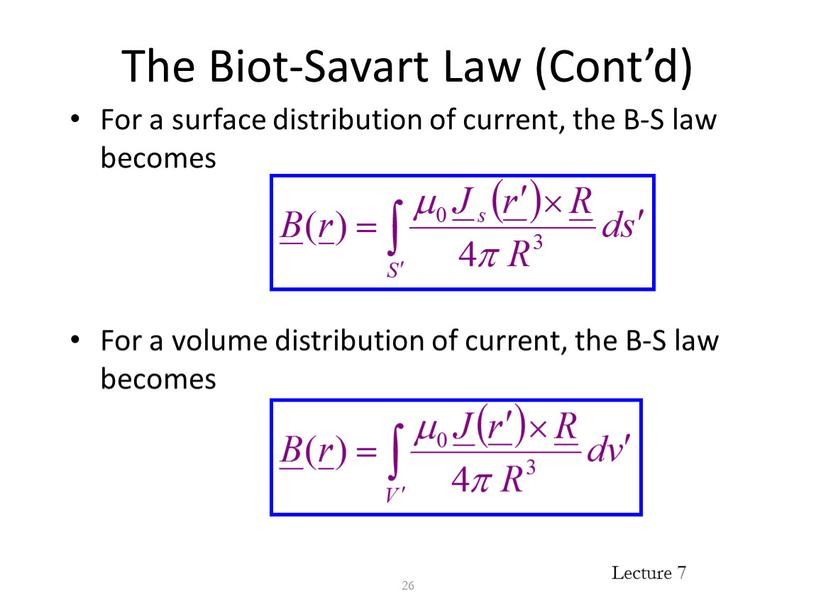The Biot-Savart Law (Cont’d)

For a surface distribution of current, the B-S law becomes

For a volume distribution of current, the B-S law becomes

26

Ampere’s Circuital Law in Integral Form

Ampere’s Circuital Law in integral form states that “the circulation of the magnetic flux density in free space is proportional to the total current through the surface bounding the path over which the circulation is computed.”

27

Ampere’s Circuital Law in Integral Form (Cont’d)

28

By convention, dS is taken to be in the direction defined by the right-hand rule applied to dl.

Since volume current
density is the most
general, we can write
Iencl in this way.

Ampere’s Law and Gauss’s Law

Just as Gauss’s law follows from Coulomb’s law, so Ampere’s circuital law follows from Ampere’s force law.
Just as Gauss’s law can be used to derive the electrostatic field from symmetric charge distributions, so Ampere’s law can be used to derive the magnetostatic field from symmetric current distributions.

29

## Applications of Ampere’s Law Ampere’s law in integral form is an integral equation for the unknown magnetic flux density resulting from a given current distribution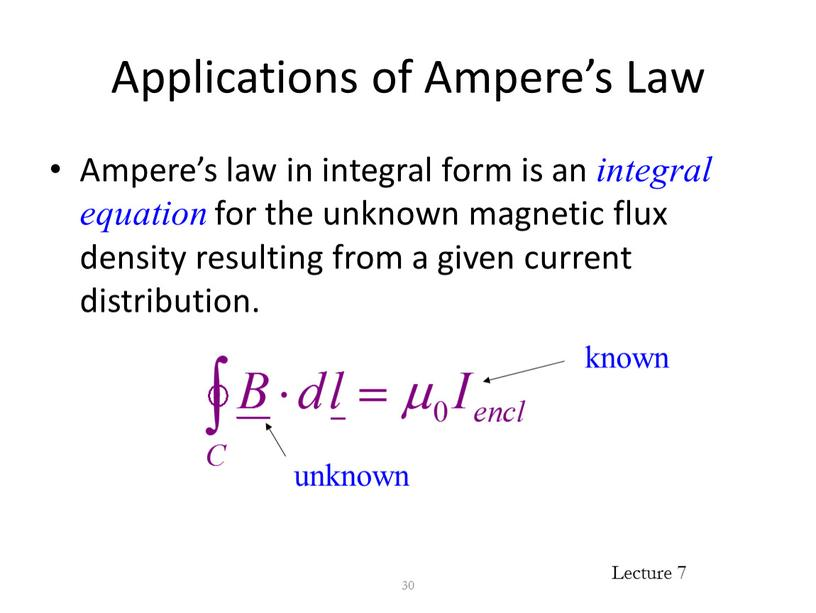Applications of Ampere’s Law

Ampere’s law in integral form is an integral equation for the unknown magnetic flux density resulting from a given current distribution.

30

known

unknown

Applications of Ampere’s Law (Cont’d)

In general, solutions to integral equations must be obtained using numerical techniques.
However, for certain symmetric current distributions closed form solutions to Ampere’s law can be obtained.

31

Applications of Ampere’s Law (Cont’d)

Closed form solution to Ampere’s law relies on our ability to construct a suitable family of Amperian paths.
An Amperian path is a closed contour to which the magnetic flux density is tangential and over which equal to a constant value.

32

Magnetic Flux Density of an Infinite Line Current Using Ampere’s Law

Consider an infinite line current along the z-axis carrying current in the +z-direction:

33

Magnetic Flux Density of an Infinite Line Current Using Ampere’s Law (Cont’d)

(1) Assume from symmetry and the right-hand rule the form of the field

(2) Construct a family of Amperian paths

34

Magnetic Flux Density of an Infinite Line Current Using Ampere’s Law (Cont’d)

(3) Evaluate the total current passing through the surface bounded by the Amperian path

35

Magnetic Flux Density of an Infinite Line Current Using Ampere’s Law (Cont’d)

36

Amperian path

Magnetic Flux Density of an Infinite Line Current Using Ampere’s Law (Cont’d)

(4) For each Amperian path, evaluate the integral

37

magnitude of B
on Amperian
path.

length
of Amperian
path.

Magnetic Flux Density of an Infinite Line Current Using Ampere’s Law (Cont’d)

(5) Solve for B on each Amperian path

38

Applying Stokes’s Theorem to Ampere’s Law

39

 Because the above must hold for any
surface S, we must have

Differential form
of Ampere’s Law

Ampere’s Law in Differential Form

Ampere’s law in differential form implies that the B-field is conservative outside of regions where current is flowing.

40

Fundamental Postulates of Magnetostatics

Ampere’s law in differential form

No isolated magnetic charges

41

B is solenoidal

## Vector Magnetic Potential Vector identity: “the divergence of the curl of any vector field is identically zero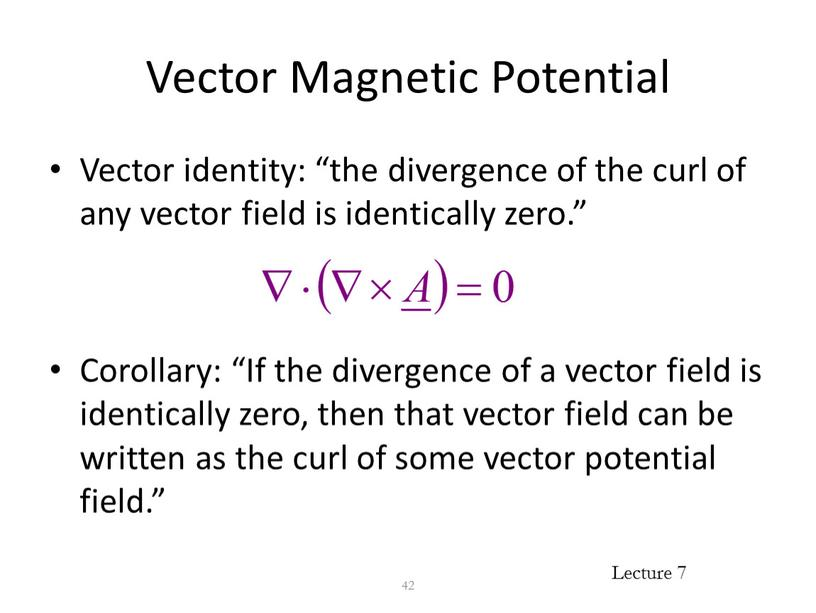Vector Magnetic Potential

Vector identity: “the divergence of the curl of any vector field is identically zero.”

Corollary: “If the divergence of a vector field is identically zero, then that vector field can be written as the curl of some vector potential field.”

42

Vector Magnetic Potential (Cont’d)

Since the magnetic flux density is solenoidal, it can be written as the curl of a vector field called the vector magnetic potential.

43

Vector Magnetic Potential (Cont’d)

The general form of the B-S law is

Note that

44

Vector Magnetic Potential (Cont’d)

Furthermore, note that the del operator operates only on the unprimed coordinates so that

45

Vector Magnetic Potential (Cont’d)

Hence, we have

46

Vector Magnetic Potential (Cont’d)

For a surface distribution of current, the vector magnetic potential is given by

For a line current, the vector magnetic potential is given by

47

Vector Magnetic Potential (Cont’d)

In some cases, it is easier to evaluate the vector magnetic potential and then use B =  A, rather than to use the B-S law to directly find B.
In some ways, the vector magnetic potential A is analogous to the scalar electric potential V.

48

Vector Magnetic Potential (Cont’d)

In classical physics, the vector magnetic potential is viewed as an auxiliary function with no physical meaning.
However, there are phenomena in quantum mechanics that suggest that the vector magnetic potential is a real (i.e., measurable) field.

49

## Magnetic Dipole A magnetic dipole comprises a small current carrying loop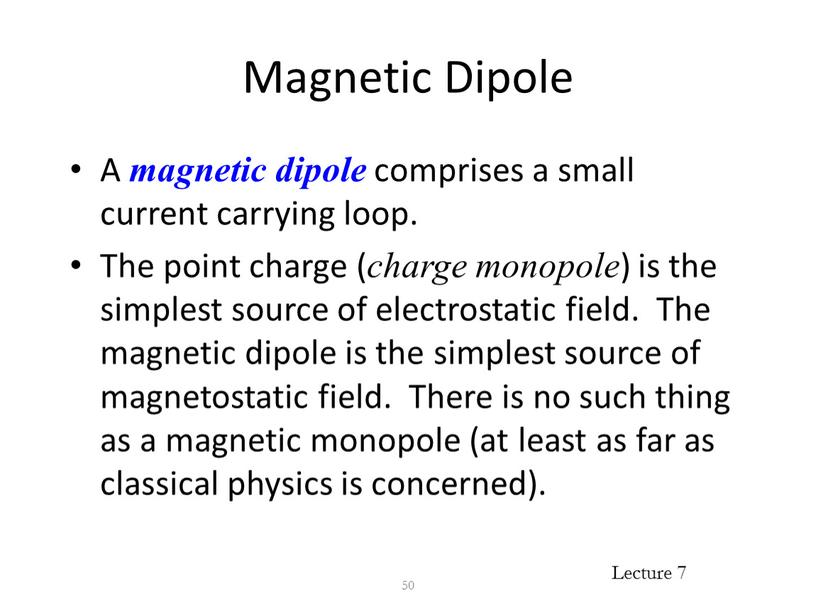Magnetic Dipole

A magnetic dipole comprises a small current carrying loop.
The point charge (charge monopole) is the simplest source of electrostatic field. The magnetic dipole is the simplest source of magnetostatic field. There is no such thing as a magnetic monopole (at least as far as classical physics is concerned).

50

## Magnetic Dipole (Cont’d) The magnetic dipole is analogous to the electric dipole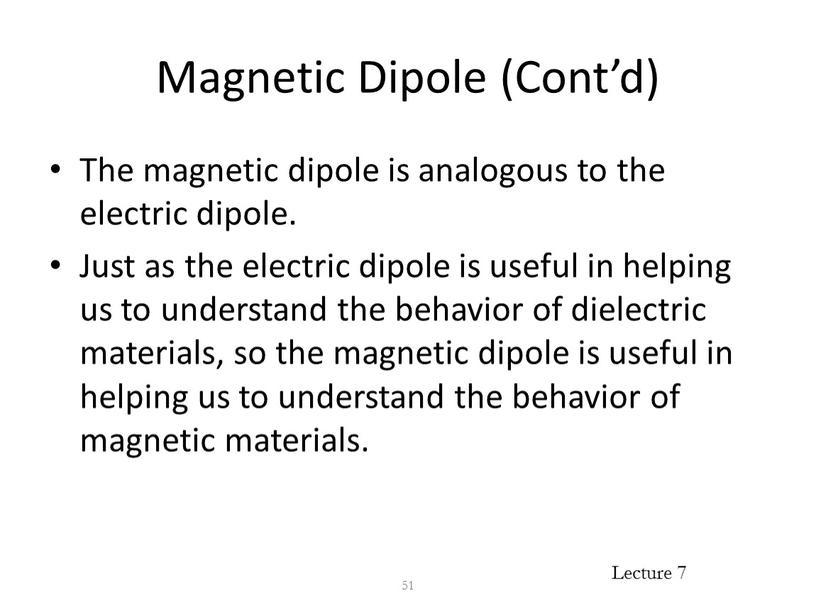Magnetic Dipole (Cont’d)

The magnetic dipole is analogous to the electric dipole.
Just as the electric dipole is useful in helping us to understand the behavior of dielectric materials, so the magnetic dipole is useful in helping us to understand the behavior of magnetic materials.

51

## Magnetic Dipole (Cont’d) Consider a small circular loop of radius b carrying a steady current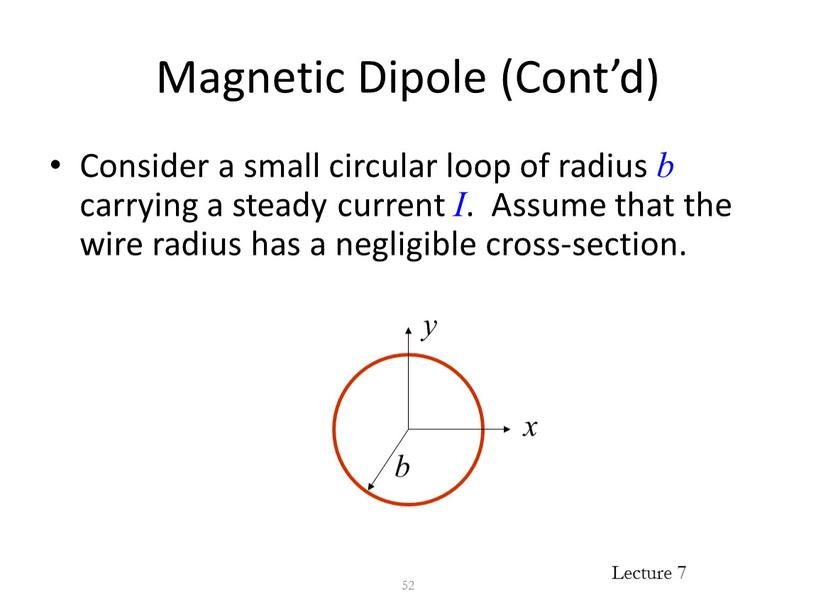Magnetic Dipole (Cont’d)

Consider a small circular loop of radius b carrying a steady current I. Assume that the wire radius has a negligible cross-section.

52

## Magnetic Dipole (Cont’d) The vector magnetic potential is evaluated for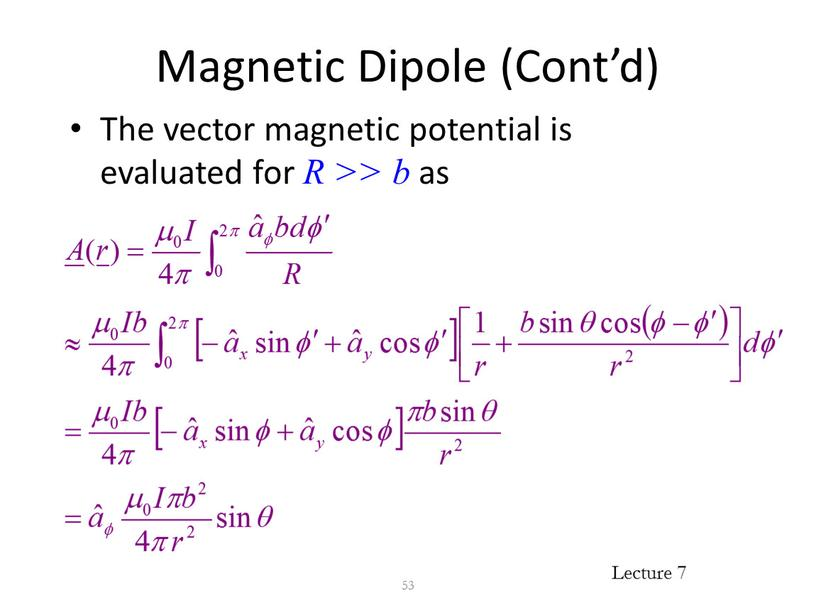Magnetic Dipole (Cont’d)

The vector magnetic potential is evaluated for R >> b as

53

## Magnetic Dipole (Cont’d) The magnetic flux density is evaluated for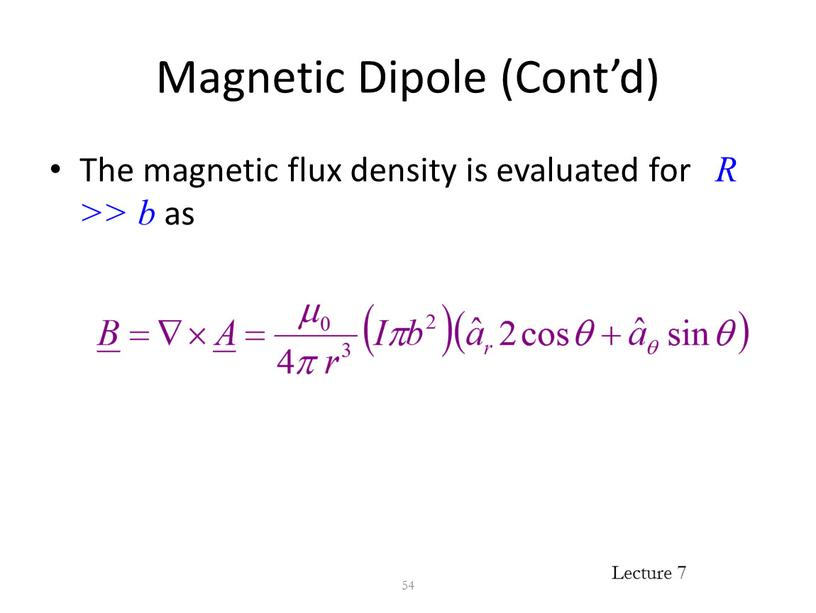Magnetic Dipole (Cont’d)

The magnetic flux density is evaluated for R >> b as

54

Magnetic Dipole (Cont’d)

Recall electric dipole

The electric field due to the electric charge dipole and the magnetic field due to the magnetic dipole are dual quantities.

55

## Magnetic Dipole Moment The magnetic dipole moment can be defined as 56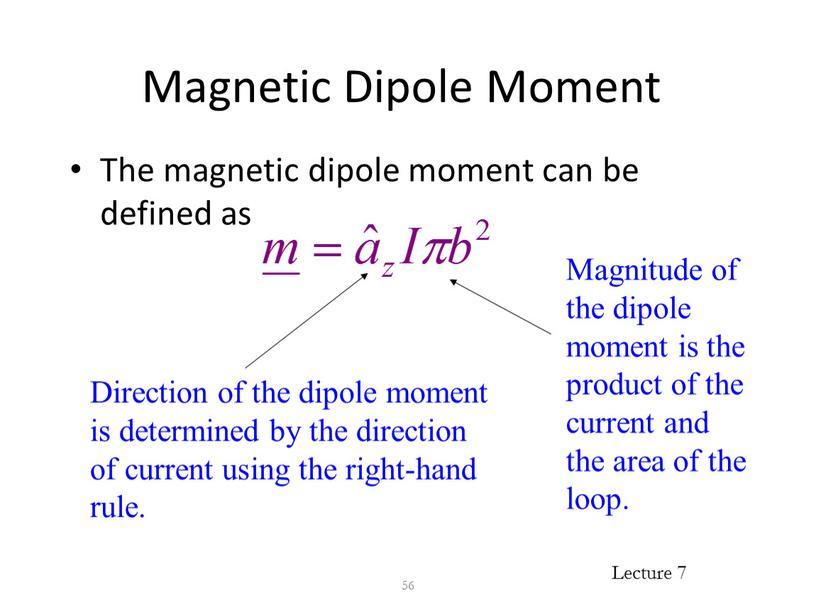Magnetic Dipole Moment

The magnetic dipole moment can be defined as

56

Direction of the dipole moment is determined by the direction of current using the right-hand rule.

Magnitude of the dipole moment is the product of the current and the area of the loop.

Magnetic Dipole Moment (Cont’d)

We can write the vector magnetic potential in terms of the magnetic dipole moment as

We can write the B field in terms of the magnetic dipole moment as

57

## Divergence of B -Field The B-field is solenoidal , i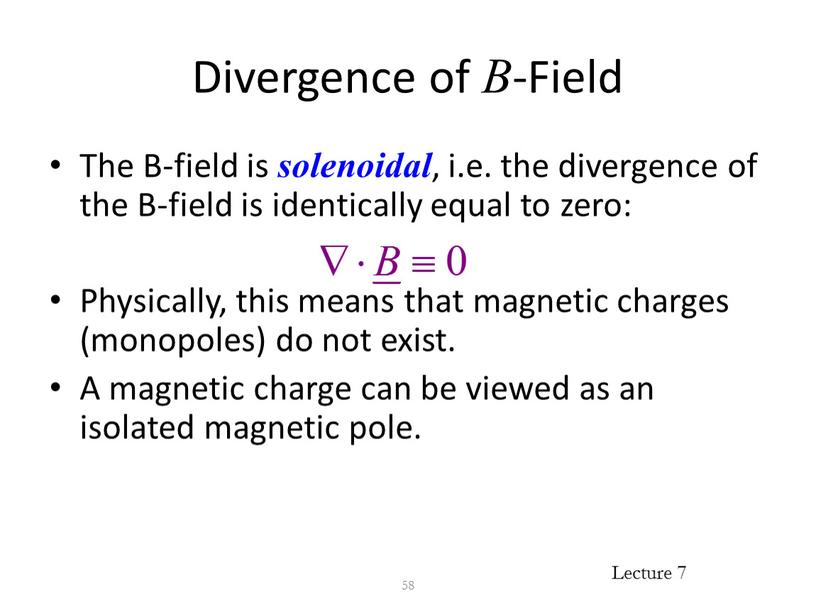Divergence of B-Field

The B-field is solenoidal, i.e. the divergence of the B-field is identically equal to zero:

Physically, this means that magnetic charges (monopoles) do not exist.
A magnetic charge can be viewed as an isolated magnetic pole.

58

Divergence of B-Field (Cont’d)

No matter how small the magnetic is divided, it always has a north pole and a south pole.
The elementary source of magnetic field is a magnetic dipole.

59

## Magnetic Flux The magnetic flux crossing an open surface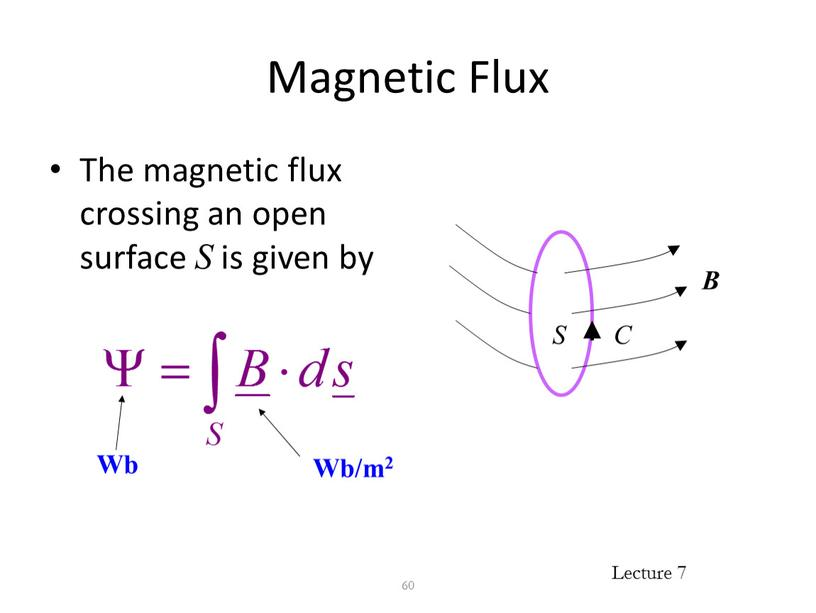Magnetic Flux

The magnetic flux crossing an open surface S is given by

60

Wb/m2

Wb

## Magnetic Flux (Cont’d) From the divergence theorem, we have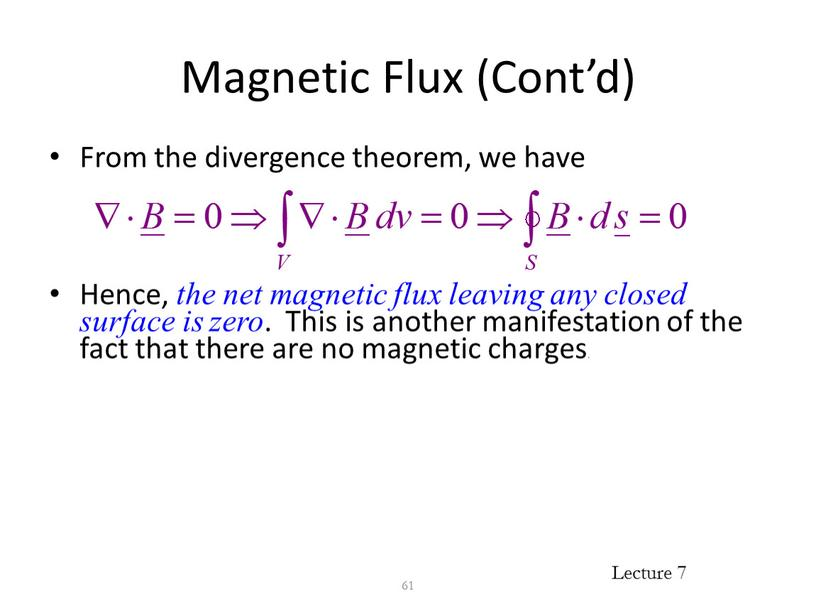Magnetic Flux (Cont’d)

From the divergence theorem, we have

Hence, the net magnetic flux leaving any closed surface is zero. This is another manifestation of the fact that there are no magnetic charges.

61

Magnetic Flux and Vector Magnetic Potential

The magnetic flux across an open surface may be evaluated in terms of the vector magnetic potential using Stokes’s theorem:

62

Electromagnetic Force

The first term in the Lorentz Force Equation represents the electric force Fe acting on a charge q within an electric field is given by.

The electromagnetic force is given by Lorentz Force Equation (After Dutch physicist Hendrik Antoon Lorentz (1853 – 1928))

The electric force is in the direction of the electric field.

The Lorentz force equation is quite useful in determining the paths charged particles will take as they move through electric and magnetic fields. If we also know the particle mass, m, the force is related to acceleration by the equation

## Since the magnetic force is at right angles to the magnetic field, the work done by the magnetic field is given by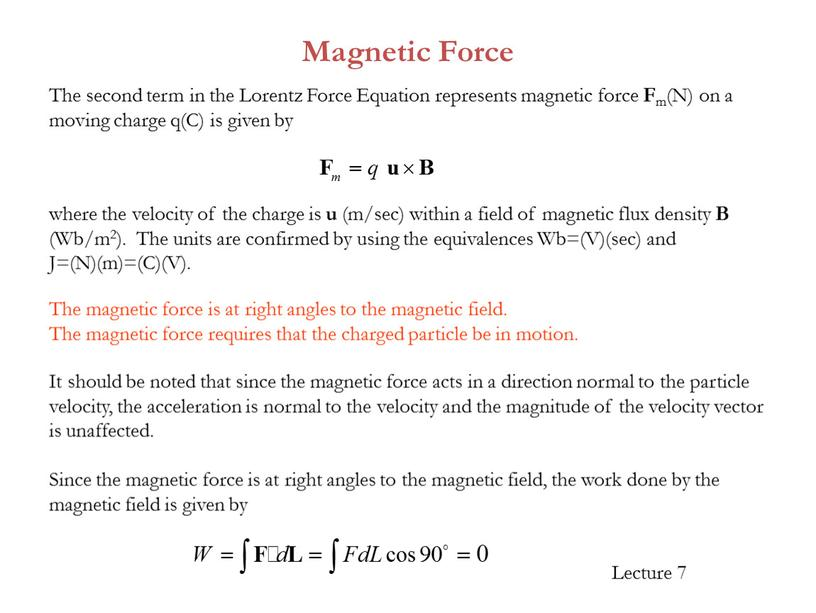Since the magnetic force is at right angles to the magnetic field, the work done by the magnetic field is given by

Magnetic Force

The magnetic force is at right angles to the magnetic field.
The magnetic force requires that the charged particle be in motion.

It should be noted that since the magnetic force acts in a direction normal to the particle velocity, the acceleration is normal to the velocity and the magnitude of the velocity vector is unaffected.

The second term in the Lorentz Force Equation represents magnetic force Fm(N) on a moving charge q(C) is given by

where the velocity of the charge is u (m/sec) within a field of magnetic flux density B (Wb/m2). The units are confirmed by using the equivalences Wb=(V)(sec) and J=(N)(m)=(C)(V).

## Magnetic Force D3.10: At a particular instant in time, in a region of space where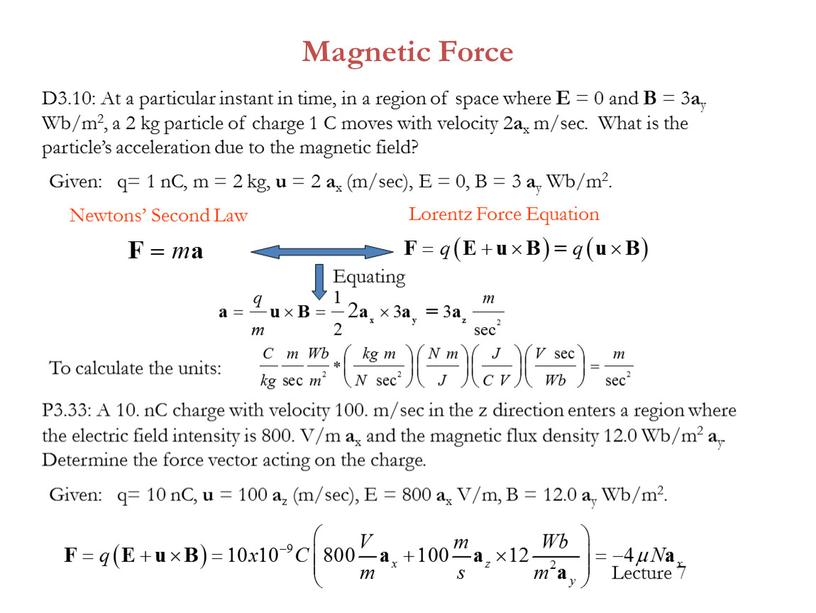Magnetic Force

D3.10: At a particular instant in time, in a region of space where E = 0 and B = 3ay Wb/m2, a 2 kg particle of charge 1 C moves with velocity 2ax m/sec. What is the particle’s acceleration due to the magnetic field?

To calculate the units:

P3.33: A 10. nC charge with velocity 100. m/sec in the z direction enters a region where the electric field intensity is 800. V/m ax and the magnetic flux density 12.0 Wb/m2 ay. Determine the force vector acting on the charge.

Given:

q= 10 nC, u = 100 az (m/sec), E = 800 ax V/m, B = 12.0 ay Wb/m2.

Given:

q= 1 nC, m = 2 kg, u = 2 ax (m/sec), E = 0, B = 3 ay Wb/m2.

Newtons’ Second Law

Lorentz Force Equation

Equating

Magnetic Force on a current Element

Consider a line conducting current in the presence of a magnetic field. We wish to find the resulting force on the line. We can look at a small, differential segment dQ of charge moving with velocity u, and can calculate the differential force on this charge from

The velocity can also be written

Therefore

Now, since dQ/dt (in C/sec) corresponds to the current I in the line, we have

(often referred to as the motor equation)

We can use to find the force from a collection of current elements, using the integral

segment

velocity

Magnetic Force – An infinite current Element

Let’s consider a line of current I in the +az direction on the z-axis. For current element IdLa, we have

The magnetic flux density B1 for an infinite length line of current is

We know this element produces magnetic field, but the field cannot exert magnetic force on the element producing it. As an analogy, consider that the electric field of a point charge can exert no electric force on itself.

What about the field from a second current element IdLb on this line?
From Biot-Savart’s Law, we see that the cross product in this particular case will be zero, since IdL and aR will be in the same direction. So, we can say that a straight line of current exerts no magnetic force on itself.

Magnetic Force – Two current Elements

By inspection of the figure we see that ρ = y and a = -ax. Inserting this in the above equation and considering that dL2 = dzaz, we have

Now let us consider a second line of current parallel to the first.
The force dF12 from the magnetic field of line 1 acting on a differential section of line 2 is

The magnetic flux density B1 for an infinite length line of current is recalled from equation to be

Magnetic Force on a current Element

To find the total force on a length L of line 2 from the field of line 1, we must integrate dF12 from +L to 0. We are integrating in this direction to account for the direction of the current.

This gives us a repulsive force.

Had we instead been seeking F21, the magnetic force acting on line 1 from the field of line 2, we would have found F21 = -F12.

Conclusion:
1) Two parallel lines with current in opposite directions experience a force of repulsion.
2) For a pair of parallel lines with current in the same direction, a force of attraction would result.

Magnetic Force on a current Element

In the more general case where the two lines are not parallel, or not straight, we could use the Law of Biot-Savart to find B1 and arrive at

This equation is known as Ampere’s Law of Force between a pair of current carrying circuits and is analogous to Coulomb’s law of force between a pair of charges.

## Magnetic Force D3.11: A pair of parallel infinite length lines each carry current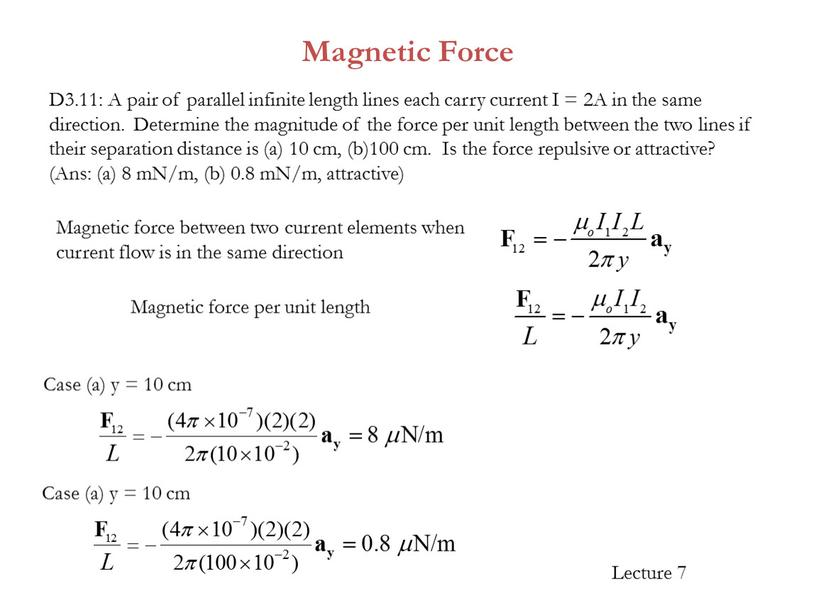Magnetic Force

D3.11: A pair of parallel infinite length lines each carry current I = 2A in the same direction. Determine the magnitude of the force per unit length between the two lines if their separation distance is (a) 10 cm, (b)100 cm. Is the force repulsive or attractive? (Ans: (a) 8 mN/m, (b) 0.8 mN/m, attractive)

Case (a) y = 10 cm

Magnetic force between two current elements when current flow is in the same direction

Magnetic force per unit length

Case (a) y = 10 cm

Magnetic Materials and Boundary Conditions

Magnetic Materials

 Material mr Diamagnetic bismuthgoldsilvercopperwater 0.999830.999860.999980.9999910.999991 Paramagnetic airaluminumplatinum 1.00000041.000021.0003 Ferromagnetic(nonlinear) cobaltnickeliron (99.8% pure)iron (99.96% pure)Mo/Ni superalloy 2506005000280,0001,000,000

The degree to which a material can influence the magnetic field is given by its relative permeability,r, analogous to relative permittivity r for dielectrics.

In free space (a vacuum), r = 1 and there is no effect on the field.

We know that current through a coil of wire will produce a magnetic field akin to that of a bar magnet.

We also know that we can greatly enhance the field by wrapping the wire around an iron core. The iron is considered a magnetic material since it can influence, in this case amplify, the magnetic field.

Relative permeabilities for a variety of materials.

## In the presence of an external magnetic field, a magnetic material gets magnetized (similar to an iron core)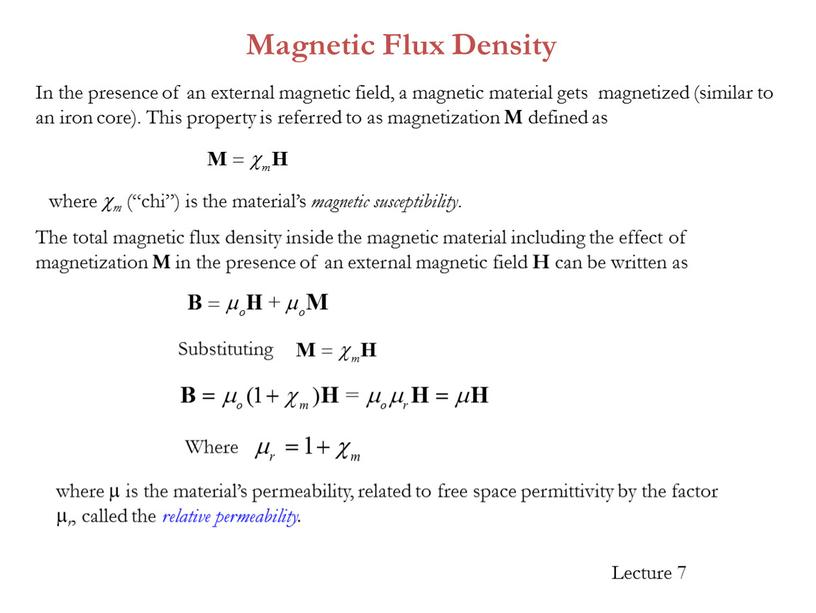In the presence of an external magnetic field, a magnetic material gets magnetized (similar to an iron core). This property is referred to as magnetization M defined as

where  is the material’s permeability, related to free space permittivity by the factor r, called the relative permeability.

Magnetic Flux Density

Where

where m (“chi”) is the material’s magnetic susceptibility.

The total magnetic flux density inside the magnetic material including the effect of magnetization M in the presence of an external magnetic field H can be written as

Substituting

Magnetostatic Boundary Conditions

Will use Ampere’s circuital law and Gauss’s law to derive normal and tangential boundary conditions for magnetostatics.

Ampere’s circuit law:

The current enclosed by the path is

We can break up the circulation of H into four integrals:

Path 1

Path 3

Path 2

Path 4

Path 1:

Path 2:

Now combining our results (i.e., Path 1 + Path 2 + Path 3 + Path 4), we obtain

A more general expression for the first magnetostatic boundary condition can be written as

where a21 is a unit vector normal going from media 2 to media 1.

Magnetostatic Boundary Conditions

Path 3:

Path 4:

Equating

Tangential BC:

ACL:

## The tangential magnetic field intensity is continuous across the boundary when the surface current density is zero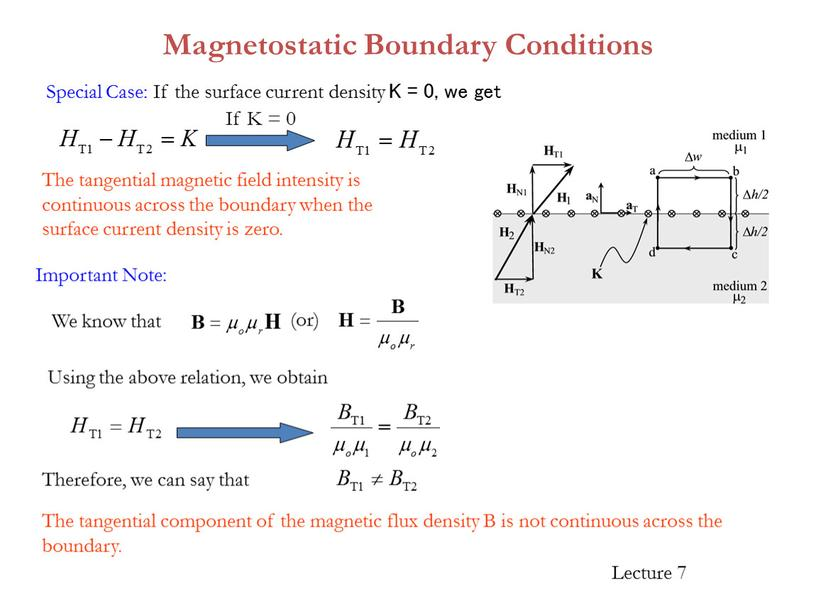The tangential magnetic field intensity is continuous across the boundary when the surface current density is zero.

We know that

Important Note:

Special Case: If the surface current density K = 0, we get

Magnetostatic Boundary Conditions

If K = 0

Using the above relation, we obtain

The tangential component of the magnetic flux density B is not continuous across the boundary.

Therefore, we can say that

(or)

Magnetostatic Boundary Conditions

Gauss’s Law for Magnetostatic fields:

To find the second boundary condition, we center a Gaussian pillbox across the interface as shown in Figure.

We can shrink h such that the flux out of the side of the pillbox is negligible. Then we have

Normal BC:

Magnetostatic Boundary Conditions

Thus, we see that the normal component of the magnetic flux density must be continuous across the boundary.

We know that

Important Note:

Using the above relation, we obtain

The normal component of the magnetic field intensity is not continuous across the boundary (but the magnetic flux density is continuous).

Therefore, we can say that

Normal BC:

Magnetostatic Boundary Conditions

Example 3.11: The magnetic field intensity is given as H1 = 6ax + 2ay + 3az (A/m) in a medium with r1 = 6000 that exists for z < 0. We want to find H2 in a medium with r2 = 3000 for z >0.

Step (a) and (b): The first step is to break H1 into its normal component (a) and its tangential component (b).
Step (c): With no current at the interface, the tangential component is the same on both sides of the boundary.
Step (d): Next, we find BN1 by multiplying HN1 by the permeability in medium 1.
Step (e): This normal component B is the same on both sides of the boundary.
Step (f): Then we can find HN2 by dividing BN2 by the permeability of medium 2.
Step (g): The last step is to sum the fields .

Magnetization and Permeability

M can be considered the magnetic field intensity due to the dipole moments when an external field H is applied
Hence, the total magnetic field intensity inside the material is M+H
The magnetic field density inside the material is B=mo(M+H)
But M depends on H, Define the magnetic susceptibility m . Hence M= m H
Hence, we get B=mo(m H +H) = B=moH (m +1)
Finally define the relative permeability mr = (m +1)
B=mo mr H= m H

81

The Nature of Magnetic Materials

Materials have a different behavior in magnetic fields
Accurate description requires quantum theory
However, simple atomic model (central nucleus surrounded by electrons) is enough for us
We can also say that B tries to make the Magnetic Dipole Moment m m in the same direction of B

82

Magnetic Dipole Moments in Atom

There are 3 magnetic dipole moments:
Moment due to rotation of the electrons
Moment due to the spin of the electrons
Moment due to the spin of the nucleus
The 3 rotations are 3 loop currents
The first two are much more effective
Electron spin is in pairs, in two opposite direction
Hence, a net moment due to electron spin occurs only when there is an un-filled shell (or orbit)
The combination of moment decide the magnetic characteristics of the material

83

Type of Magnetic Materials

We will study 6 types:
Diamagnetic
Paramagnetic
Ferromagnetic
Anti-ferromagnetic
Ferrimagnetic
Super paramagnetic

84

## Diamagnetic Materials Without an external magnetic field, diamagnetic materials have no net magnetic field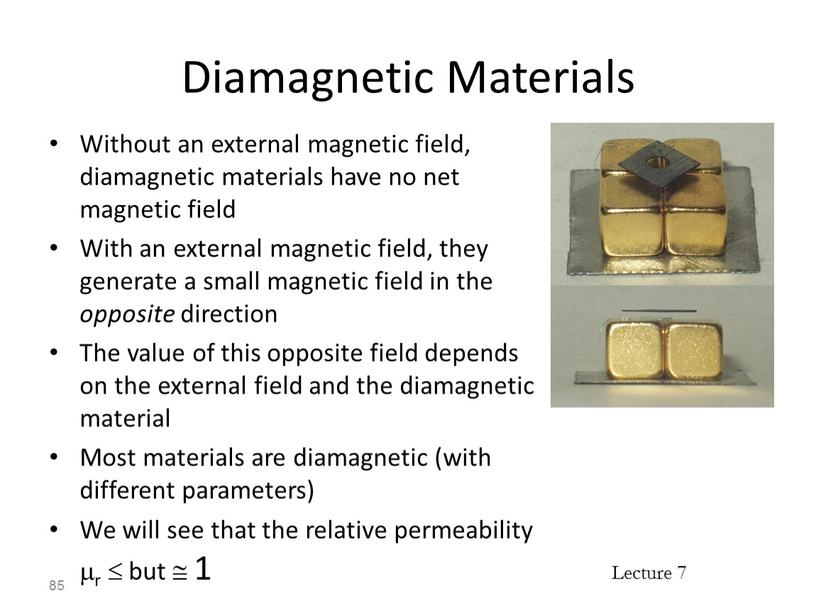Diamagnetic Materials

Without an external magnetic field, diamagnetic materials have no net magnetic field
With an external magnetic field, they generate a small magnetic field in the opposite direction
The value of this opposite field depends on the external field and the diamagnetic material
Most materials are diamagnetic (with different parameters)
We will see that the relative permeability mr  but  1

85

## Why Diamagnetic Materials, 1 Each atom has zero total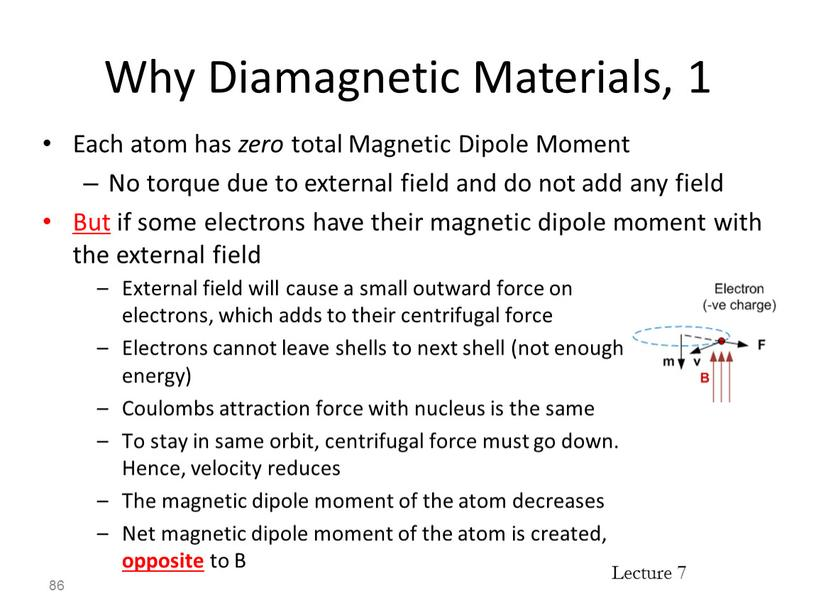Why Diamagnetic Materials, 1

Each atom has zero total Magnetic Dipole Moment
No torque due to external field and do not add any field
But if some electrons have their magnetic dipole moment with the external field

86

External field will cause a small outward force on electrons, which adds to their centrifugal force
Electrons cannot leave shells to next shell (not enough energy)
Coulombs attraction force with nucleus is the same
To stay in same orbit, centrifugal force must go down. Hence, velocity reduces
The magnetic dipole moment of the atom decreases
Net magnetic dipole moment of the atom is created, opposite to B

## Why Diamagnetic Materials, 2 Also if some electrons have their magnetic dipole moment opposite to the external field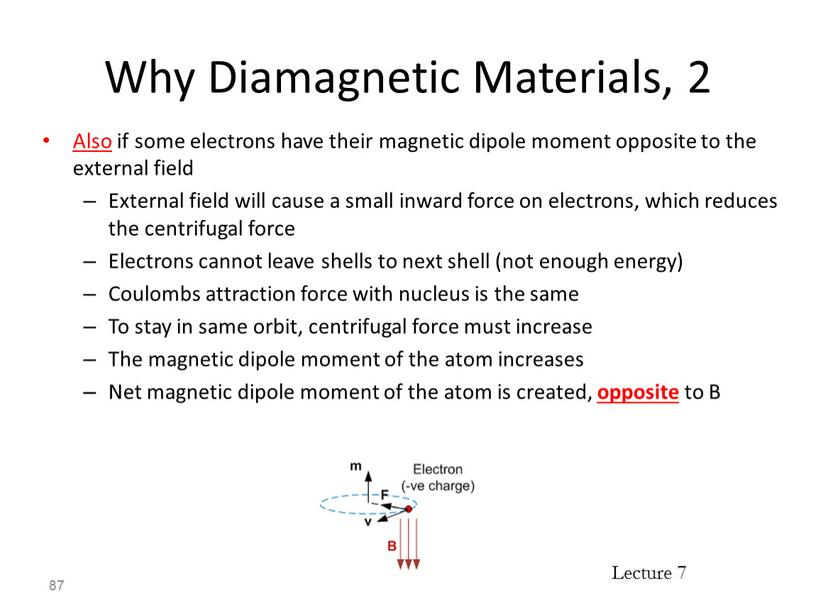Why Diamagnetic Materials, 2

Also if some electrons have their magnetic dipole moment opposite to the external field
External field will cause a small inward force on electrons, which reduces the centrifugal force
Electrons cannot leave shells to next shell (not enough energy)
Coulombs attraction force with nucleus is the same
To stay in same orbit, centrifugal force must increase
The magnetic dipole moment of the atom increases
Net magnetic dipole moment of the atom is created, opposite to B

87

## Diamagnetic Materials Note that mr = 1 + susceptibility 88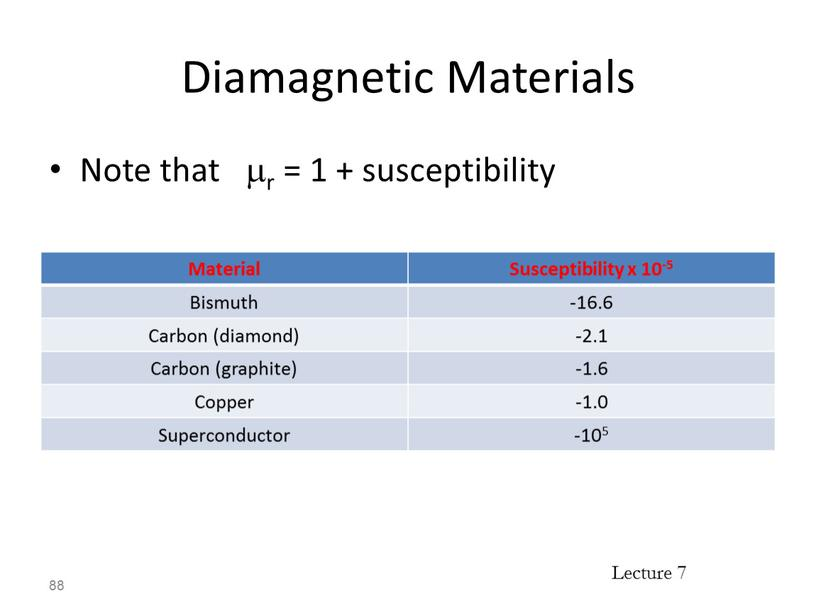Diamagnetic Materials

Note that mr = 1 + susceptibility

88

 Material Susceptibility x 10-5 Bismuth -16.6 Carbon (diamond) -2.1 Carbon (graphite) -1.6 Copper -1.0 Superconductor -105

## Paramagnetic Materials Atoms have a small net magnetic dipole moment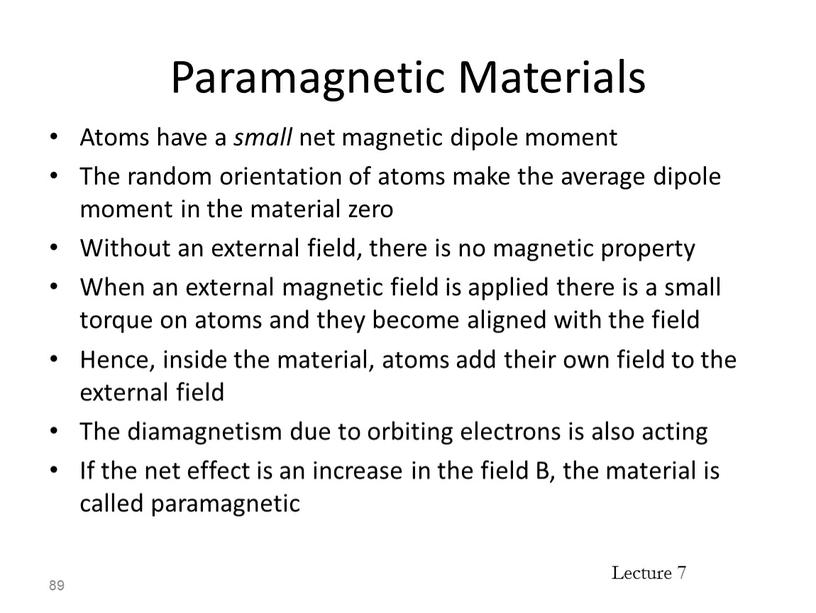Paramagnetic Materials

Atoms have a small net magnetic dipole moment
The random orientation of atoms make the average dipole moment in the material zero
Without an external field, there is no magnetic property
When an external magnetic field is applied there is a small torque on atoms and they become aligned with the field
Hence, inside the material, atoms add their own field to the external field
The diamagnetism due to orbiting electrons is also acting
If the net effect is an increase in the field B, the material is called paramagnetic

89

## Paramagnetic Materials Note that mr = 1 + susceptibility 90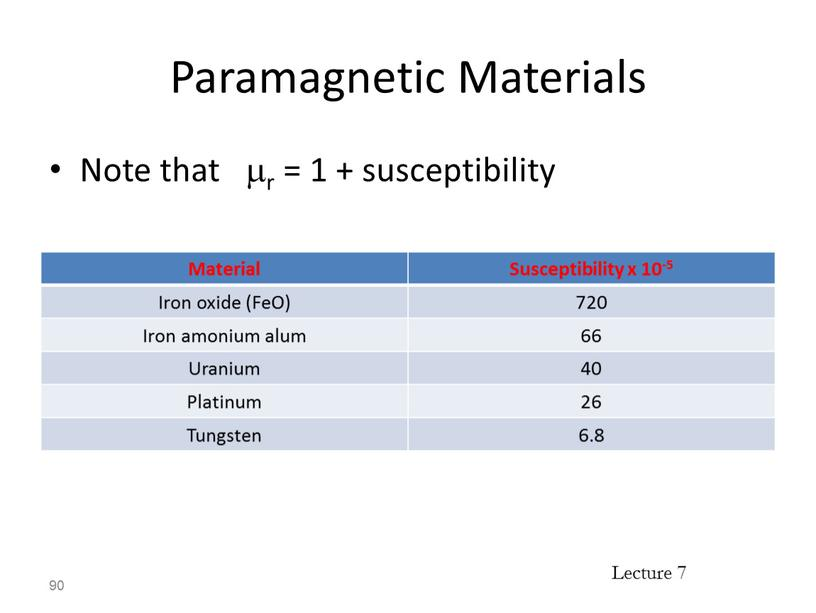Paramagnetic Materials

Note that mr = 1 + susceptibility

90

 Material Susceptibility x 10-5 Iron oxide (FeO) 720 Iron amonium alum 66 Uranium 40 Platinum 26 Tungsten 6.8

## Ferromagnetic Materials Atoms have large dipole moment, they affect each other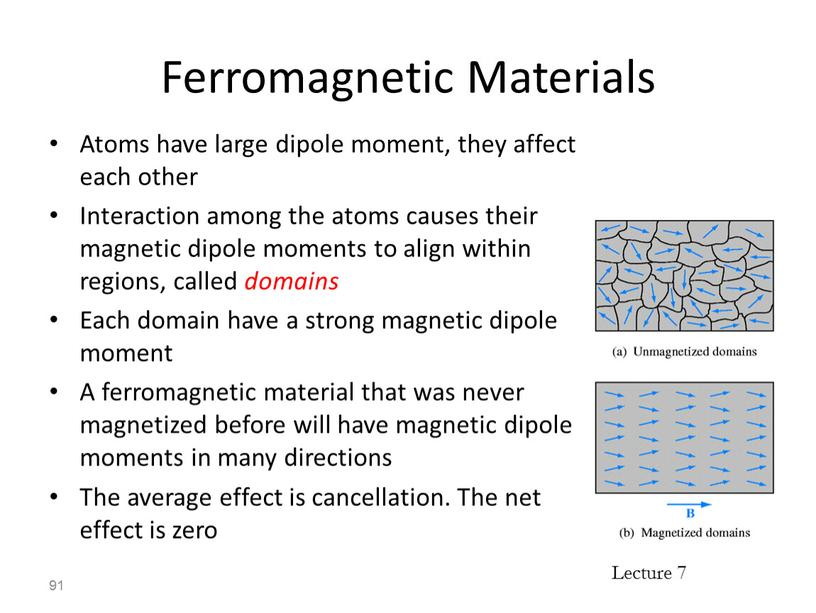Ferromagnetic Materials

Atoms have large dipole moment, they affect each other
Interaction among the atoms causes their magnetic dipole moments to align within regions, called domains
Each domain have a strong magnetic dipole moment
A ferromagnetic material that was never magnetized before will have magnetic dipole moments in many directions
The average effect is cancellation. The net effect is zero

91

## Ferromagnetic Materials When an external magnetic field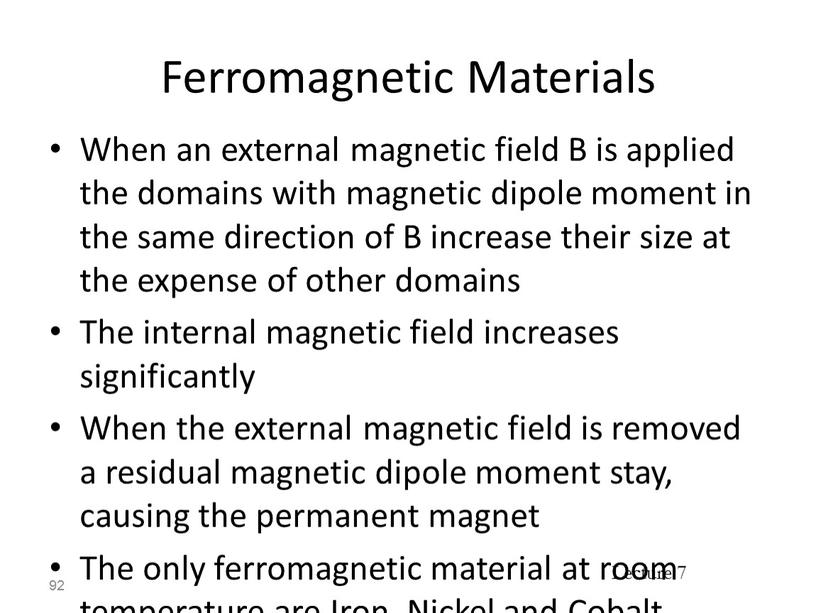Ferromagnetic Materials

When an external magnetic field B is applied the domains with magnetic dipole moment in the same direction of B increase their size at the expense of other domains
The internal magnetic field increases significantly
When the external magnetic field is removed a residual magnetic dipole moment stay, causing the permanent magnet
The only ferromagnetic material at room temperature are Iron, Nickel and Cobalt
They loose ferromagnetism at temperature > Curie temperature (which is 770o C for iron)

92

Ferromagnetic Materials

Curie Temperatures:
Iron: 770oC
Nickel: 354o C
Cobalt: 1115o C

93

 Medium Relative Permeability μr Mu Metal 20,000 Permalloy 8000 Electrical Steel 4000 Ferrite (nickel zinc) 16-640 Ferrite (manganese zinc) >640 Steel 100 Nickel 100-600

## Antiferromagnetic Materials Atoms have a net dipole moment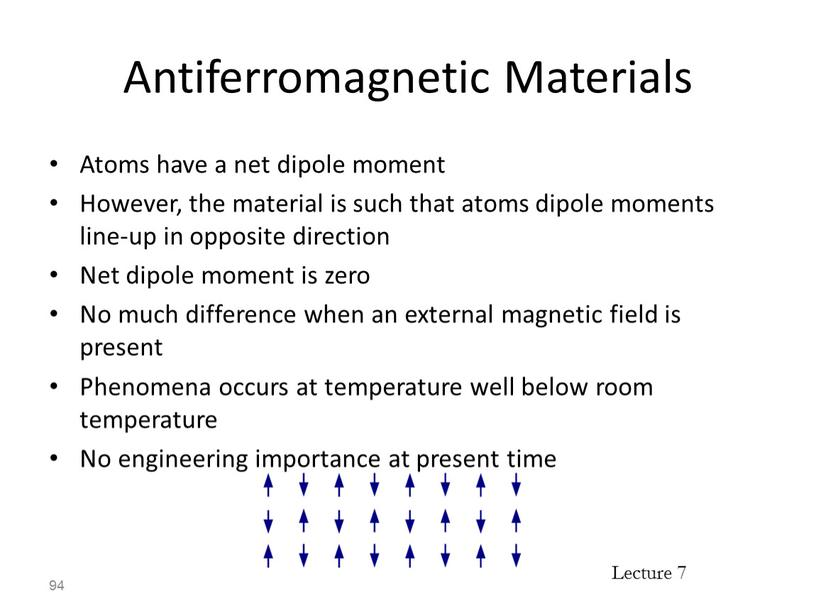Antiferromagnetic Materials

Atoms have a net dipole moment
However, the material is such that atoms dipole moments line-up in opposite direction
Net dipole moment is zero
No much difference when an external magnetic field is present
Phenomena occurs at temperature well below room temperature
No engineering importance at present time

94

## Ferrimagnetic Materials Similar to antiferromagnetic materials, atoms dipole moments line-up in opposite direction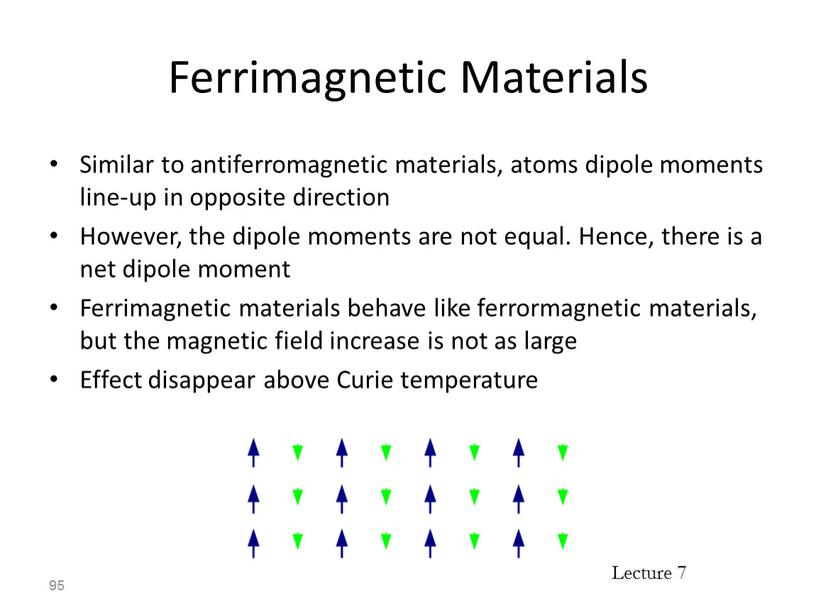Ferrimagnetic Materials

Similar to antiferromagnetic materials, atoms dipole moments line-up in opposite direction
However, the dipole moments are not equal. Hence, there is a net dipole moment
Ferrimagnetic materials behave like ferrormagnetic materials, but the magnetic field increase is not as large
Effect disappear above Curie temperature

95

## Ferrimagnetic Materials The main advantage is that they have high resistance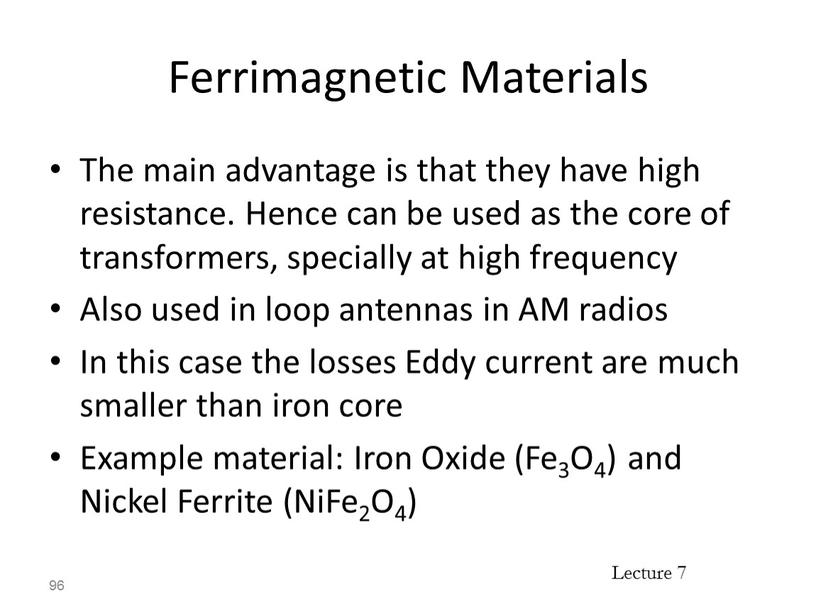Ferrimagnetic Materials

The main advantage is that they have high resistance. Hence can be used as the core of transformers, specially at high frequency
Also used in loop antennas in AM radios
In this case the losses Eddy current are much smaller than iron core
Example material: Iron Oxide (Fe3O4) and Nickel Ferrite (NiFe2O4)

96

Super Paramagnetic Materials

Composed of Ferromagnetic particles inside non-ferromagnetic materials
Domains occur but can not expand when exposed to external field
Used in magnetic tapes for audio and video tape recording

97

Magnetization and Permeability

Now let us discuss the magnetic effect of magnetic material in a quantitative manner
Let us call the current inside the material due to electron orbit, electron spin and atom spin by the bound current Ib
The material includes many dipole moments m (units A m2) that add-up
Define the Magnetization M as the magnetic dipole moment per unit volume
M has a unit A/m (which is similar to the units of H)

98

Скачать файл